Authors: Koki Tsuyuzaki [aut, cre]
Compiled: Tue Apr 26 16:16:57 2022

# 1 Setting

``````suppressPackageStartupMessages(library("DelayedTensor"))
suppressPackageStartupMessages(library("DelayedArray"))
suppressPackageStartupMessages(library("HDF5Array"))
suppressPackageStartupMessages(library("DelayedRandomArray"))

darr1 <- RandomUnifArray(c(2,3,4))
darr2 <- RandomUnifArray(c(2,3,4))``````

There are several settings in DelayedTensor.

First, the sparsity of the intermediate DelayedArray objects calculated inside DelayedTensor is set by `setSparse`.

Note that the sparse mode is experimental.

Whether it contributes to higher speed and lower memory is quite dependent on the sparsity of the DelayedArray, and the current implementation does not recognize the block size, which may cause out-of-memory errors, when the data is extremely huge.

Here, we specify `as.sparse` as `FALSE` (this is also the default value for now).

``DelayedTensor::setSparse(as.sparse=FALSE)``

Next, the verbose message is suppressed by `setVerbose`. This is useful when we want to monitor the calculation process.

Here we specify `as.verbose` as `FALSE` (this is also the default value for now).

``DelayedTensor::setVerbose(as.verbose=FALSE)``

The block size of block processing is specified by `setAutoBlockSize`. When the sparse mode is off, all the functions of DelayedTensor are performed as block processing, in which each block vector/matrix/tensor is expanded to memory space from on-disk file incrementally so as not to exceed the specified size.

Here, we specify the block size as `1E+8`.

``setAutoBlockSize(size=1E+8)``
``## automatic block size set to 1e+08 bytes (was 1e+08)``

Finally, the temporal directory to store the intermediate HDF5 files during running DelayedTensor is specified by `setHDF5DumpDir`.

Note that in many systems the `/var` directory has the storage limitation, so if there is no enough space, user should specify the other directory.

``````# tmpdir <- paste(sample(c(letters,1:9), 10), collapse="")
# dir.create(tmpdir, recursive=TRUE))
tmpdir <- tempdir()
setHDF5DumpDir(tmpdir)``````

These specified values are also extracted by each getter function.

``DelayedTensor::getSparse()``
``````## \$delayedtensor.sparse
##  FALSE``````
``DelayedTensor::getVerbose()``
``````## \$delayedtensor.verbose
##  FALSE``````
``getAutoBlockSize()``
``##  1e+08``
``getHDF5DumpDir()``
``##  "/tmp/Rtmplp0rxi"``

# 2 Tensor Arithmetic Operations

## 2.1 Unfold/Fold Operations

Unfold (a.k.a. matricizing) operations are used to reshape a tensor into a matrix.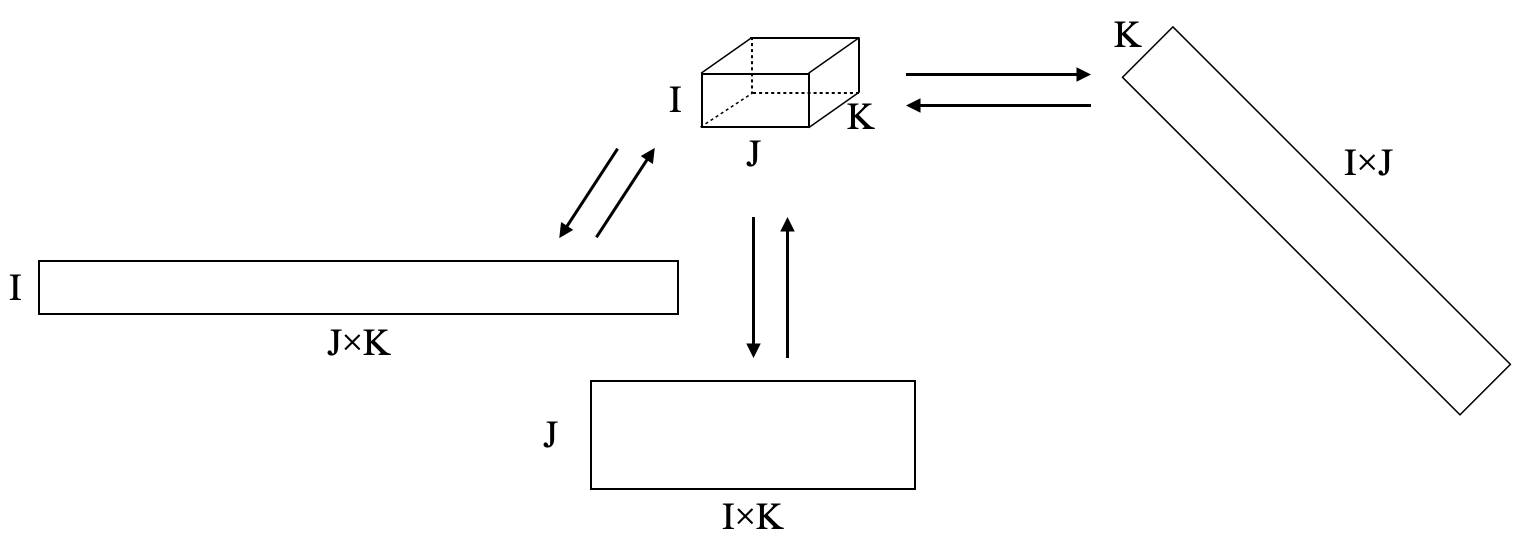Figure 1: Unfold/Fold Operasions

In `unfold`, `row_idx` and `col_idx` are specified to set which modes are used as the row/column.

``````dmat1 <- DelayedTensor::unfold(darr1, row_idx=c(1,2), col_idx=3)
dmat1``````
``````## <6 x 4> matrix of class HDF5Matrix and type "double":
##            [,1]       [,2]       [,3]       [,4]
## [1,] 0.91409958 0.78113263 0.07017729 0.64063279
## [2,] 0.08632737 0.86869108 0.58917277 0.30181345
## [3,] 0.76137827 0.69378194 0.99163780 0.66496589
## [4,] 0.43164016 0.65569837 0.58887649 0.49547227
## [5,] 0.37329117 0.09482776 0.38479712 0.71593550
## [6,] 0.92093945 0.17523761 0.14463644 0.32093605``````

`fold` is the inverse operation of `unfold`, which is used to reshape a matrix into a tensor.

In `fold`, `row_idx`/`col_idx` are specified to set which modes correspond the row/column of the output tensor and `modes` is specified to set the mode of the output tensor.

``````dmat1_to_darr1 <- DelayedTensor::fold(dmat1,
row_idx=c(1,2), col_idx=3, modes=dim(darr1))
dmat1_to_darr1``````
``````## <2 x 3 x 4> array of class DelayedArray and type "double":
## ,,1
##            [,1]       [,2]       [,3]
## [1,] 0.91409958 0.76137827 0.37329117
## [2,] 0.08632737 0.43164016 0.92093945
##
## ,,2
##            [,1]       [,2]       [,3]
## [1,] 0.78113263 0.69378194 0.09482776
## [2,] 0.86869108 0.65569837 0.17523761
##
## ,,3
##            [,1]       [,2]       [,3]
## [1,] 0.07017729 0.99163780 0.38479712
## [2,] 0.58917277 0.58887649 0.14463644
##
## ,,4
##           [,1]      [,2]      [,3]
## [1,] 0.6406328 0.6649659 0.7159355
## [2,] 0.3018134 0.4954723 0.3209361``````
``identical(as.array(darr1), as.array(dmat1_to_darr1))``
``##  TRUE``

There are some wrapper functions of `unfold` and `fold`.

For example, in `k_unfold`, mode `m` is used as the row, and the other modes are is used as the column.

`k_fold` is the inverse operation of `k_unfold`.

``````dmat2 <- DelayedTensor::k_unfold(darr1, m=1)
dmat2_to_darr1 <- k_fold(dmat2, m=1, modes=dim(darr1))
identical(as.array(darr1), as.array(dmat2_to_darr1))``````
``##  TRUE``
``````dmat3 <- DelayedTensor::k_unfold(darr1, m=2)
dmat3_to_darr1 <- k_fold(dmat3, m=2, modes=dim(darr1))
identical(as.array(darr1), as.array(dmat3_to_darr1))``````
``##  TRUE``
``````dmat4 <- DelayedTensor::k_unfold(darr1, m=3)
dmat4_to_darr1 <- k_fold(dmat4, m=3, modes=dim(darr1))
identical(as.array(darr1), as.array(dmat4_to_darr1))``````
``##  TRUE``

In `rs_unfold`, mode `m` is used as the row, and the other modes are is used as the column.

`rs_fold` and `rs_unfold` also perform the same operations.

On the other hand, `cs_unfold` specifies the mode `m` as the column and the other modes are specified as the column.

`cs_fold` is the inverse operation of `cs_unfold`.

``````dmat8 <- DelayedTensor::cs_unfold(darr1, m=1)
dmat8_to_darr1 <- DelayedTensor::cs_fold(dmat8, m=1, modes=dim(darr1))
identical(as.array(darr1), as.array(dmat8_to_darr1))``````
``##  TRUE``
``````dmat9 <- DelayedTensor::cs_unfold(darr1, m=2)
dmat9_to_darr1 <- DelayedTensor::cs_fold(dmat9, m=2, modes=dim(darr1))
identical(as.array(darr1), as.array(dmat9_to_darr1))``````
``##  TRUE``
``````dmat10 <- DelayedTensor::cs_unfold(darr1, m=3)
dmat10_to_darr1 <- DelayedTensor::cs_fold(dmat10, m=3, modes=dim(darr1))
identical(as.array(darr1), as.array(dmat10_to_darr1))``````
``##  TRUE``

In `matvec`, m=2 is specified as unfold.

`unmatvec` is the inverse operation of `matvec`.

``````dmat11 <- DelayedTensor::matvec(darr1)
dmat11_darr1 <- DelayedTensor::unmatvec(dmat11, modes=dim(darr1))
identical(as.array(darr1), as.array(dmat11_darr1))``````
``##  TRUE``

`ttm` multiplies a tensor by a matrix.

`m` specifies in which mode the matrix will be multiplied.

``````dmatZ <- RandomUnifArray(c(10,4))
DelayedTensor::ttm(darr1, dmatZ, m=3)``````
``````## <2 x 3 x 10> array of class DelayedArray and type "double":
## ,,1
##           [,1]      [,2]      [,3]
## [1,] 1.3381367 2.1534562 1.0397790
## [2,] 1.3935900 1.4997062 0.8021832
##
## ,,2
##          [,1]     [,2]     [,3]
## [1,] 1.616298 2.322020 1.180709
## [2,] 1.217502 1.538101 1.207781
##
## ,,3
##           [,1]      [,2]      [,3]
## [1,] 1.6608321 1.4765913 0.5702775
## [2,] 0.9488167 1.1008044 1.0534692
##
## ...
##
## ,,8
##           [,1]      [,2]      [,3]
## [1,] 0.3772188 0.8576583 0.3879916
## [2,] 0.4125701 0.5197007 0.3754053
##
## ,,9
##           [,1]      [,2]      [,3]
## [1,] 0.9548712 1.0683232 0.5500124
## [2,] 0.3670101 0.6615511 0.8576662
##
## ,,10
##          [,1]     [,2]     [,3]
## [1,] 1.840272 2.220438 1.113567
## [2,] 1.287769 1.553215 1.216637``````

`ttl` multiplies a tensor by multiple matrices.

`ms` specifies in which mode these matrices will be multiplied.

``````dmatX <- RandomUnifArray(c(10,2))
dmatY <- RandomUnifArray(c(10,3))
dlizt <- list(dmatX = dmatX, dmatY = dmatY)
DelayedTensor::ttl(darr1, dlizt, ms=c(1,2))``````
``````## <10 x 10 x 4> array of class DelayedArray and type "double":
## ,,1
##             [,1]       [,2]       [,3] ...       [,9]      [,10]
##  [1,]  0.5718995  1.0574146  1.5875021   .  0.9408706  1.2738833
##  [2,]  0.5686503  1.0164066  1.4345055   .  0.8915427  1.1169409
##   ...          .          .          .   .          .          .
##  [9,] 0.31101802 0.55164408 0.76702395   . 0.48225513 0.59263616
## [10,] 0.06015311 0.10584440 0.14486159   . 0.09220659 0.11099505
##
## ...
##
## ,,4
##             [,1]       [,2]       [,3] ...       [,9]      [,10]
##  [1,]  0.5136839  0.9482170  1.4476916   .  0.8742744  1.1725316
##  [2,]  0.4535844  0.8351406  1.2903483   .  0.7922945  1.0520452
##   ...          .          .          .   .          .          .
##  [9,] 0.24110759 0.44363410 0.68755129   . 0.42394239 0.56151955
## [10,] 0.04524732 0.08319437 0.12936652   . 0.08012867 0.10584569``````

## 2.2 Vectorization

`vec` collapses a DelayedArray into a 1D DelayedArray (vector).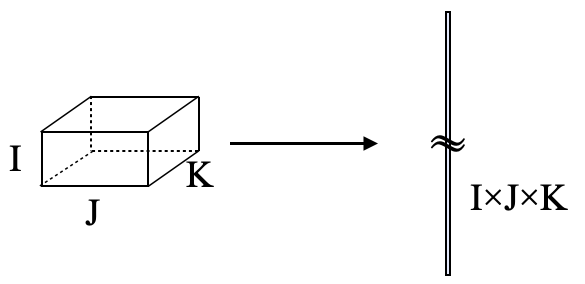Figure 2: Vectorization

``DelayedTensor::vec(darr1)``
``````## <24> array of class HDF5Array and type "double":
##                                  .              
## 0.91409958 0.08632737 0.76137827          .  0.7159355  0.3209361``````

## 2.3 Norm Operations

`fnorm` calculates the Frobenius norm of a DelayedArray.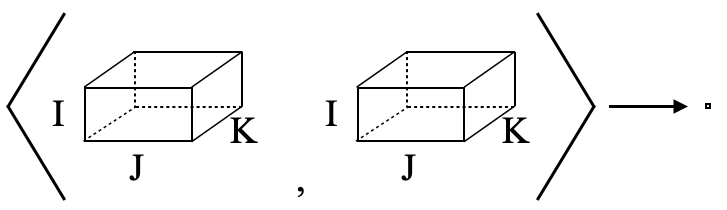Figure 3: Norm Operations

``DelayedTensor::fnorm(darr1)``
``##  2.927145``

`innerProd` calculates the inner product value of two DelayedArray.

``DelayedTensor::innerProd(darr1, darr2)``
``##  6.859228``

## 2.4 Outer Product

Inner product multiplies two tensors and collapses to 0D tensor (norm). On the other hand, the outer product is an operation that leaves all subscripts intact.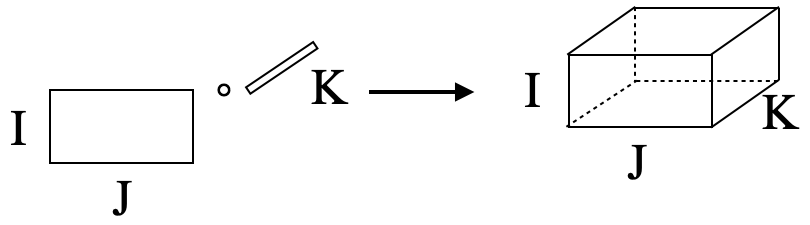Figure 4: Outer Product

``DelayedTensor::outerProd(darr1[,,1], darr2[,,1])``
``````## <2 x 3 x 2 x 3> array of class HDF5Array and type "double":
## ,,1,1
##            [,1]       [,2]       [,3]
## [1,] 0.84033895 0.69994105 0.34316952
## [2,] 0.07936143 0.39681020 0.84662689
##
## ,,2,1
##            [,1]       [,2]       [,3]
## [1,] 0.85313904 0.71060258 0.34839669
## [2,] 0.08057027 0.40285443 0.85952276
##
## ,,1,2
##            [,1]       [,2]       [,3]
## [1,] 0.90781208 0.75614124 0.37072354
## [2,] 0.08573358 0.42867119 0.91460490
##
## ,,2,2
##            [,1]       [,2]       [,3]
## [1,] 0.11688179 0.09735400 0.04773106
## [2,] 0.01103829 0.05519188 0.11775637
##
## ,,1,3
##            [,1]       [,2]       [,3]
## [1,] 0.22135545 0.18437294 0.09039500
## [2,] 0.02090476 0.10452461 0.22301177
##
## ,,2,3
##            [,1]       [,2]       [,3]
## [1,] 0.68043130 0.56674963 0.27786797
## [2,] 0.06425978 0.32130140 0.68552271``````

## 2.5 Diagonal Operations

Using `DelayedDiagonalArray`, we can originally create a diagonal DelayedArray by specifying the dimensions (modes) and the values.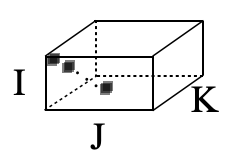Figure 5: Diagonal Operations

``````dgdarr <- DelayedTensor::DelayedDiagonalArray(c(5,6,7), 1:5)
dgdarr``````
``````## <5 x 6 x 7> sparse array of class DelayedArray and type "integer":
## ,,1
##      [,1] [,2] [,3] [,4] [,5] [,6]
## [1,]    1    0    0    0    0    0
## [2,]    0    0    0    0    0    0
## [3,]    0    0    0    0    0    0
## [4,]    0    0    0    0    0    0
## [5,]    0    0    0    0    0    0
##
## ...
##
## ,,7
##      [,1] [,2] [,3] [,4] [,5] [,6]
## [1,]    0    0    0    0    0    0
## [2,]    0    0    0    0    0    0
## [3,]    0    0    0    0    0    0
## [4,]    0    0    0    0    0    0
## [5,]    0    0    0    0    0    0``````

Similar to the `diag` of the base package, the `diag` of DelayedTensor is used to extract and assign values to DelayedArray.

``DelayedTensor::diag(dgdarr)``
``````## <5> array of class DelayedArray and type "integer":
##     
##   1   2   3   4   5``````
``````DelayedTensor::diag(dgdarr) <- c(1111, 2222, 3333, 4444, 5555)
DelayedTensor::diag(dgdarr)``````
``````## <5> array of class DelayedArray and type "double":
##          
## 1111 2222 3333 4444 5555``````

## 2.6 Mode-wise Operations

`modeSum` calculates the summation for a given mode `m` of a DelayedArray. The mode specified as `m` is collapsed into 1D as follows.